﻿ 基于非负最小二乘的矢量阵反卷积波束形成方法
«上一篇文章快速检索 高级检索

 哈尔滨工程大学学报2019, Vol. 40Issue (7): 1217-1223  DOI: 10.11990/jheu.2018110590

### 引用本文SUN Dajun, MA Chao, MEI Jidan, et al. Deconvolved conventional beamforming of a vector-sensor array based on non-negative least squares[J]. Journal of Harbin Engineering University, 2019, 40(7), 1217-1223. DOI: 10.11990/jheu.201811059.### 文章历史

1. 哈尔滨工程大学 水声技术重点实验室, 黑龙江 哈尔滨 150001;
2. 海洋信息获取与安全工信部重点实验室(哈尔滨工程大学) 工业和信息化部, 黑龙江 哈尔滨 150001;
3. 哈尔滨工程大学, 水声工程学院, 黑龙江 哈尔滨 150001

Deconvolved conventional beamforming of a vector-sensor array based on non-negative least squares
SUN Dajun 1,2,3, MA Chao 1,2,3, MEI Jidan 1,2,3, SHI Wenpei 1,2,3
1. Acoustic Science and Technology Laboratory, Harbin Engineering University, Harbin 150001, China;
2. Key Laboratory of Marine Information Acquisition and Security(Harbin Engineering University), Ministry of Industry and Information Technology, Harbin 150001, China;
3. College of Underwater Acoustic Engineering, Harbin Engineering University, Harbin 150001, China
Abstract: Aiming at the problem that the existing deconvolved beamforming method cannot be directly applied to the vector array with a shift-variant point spread function (PSF), a deconvolution solution based on non-negative least squares (NNLS) method is proposed. A generalized convolution model of a vector-sensor array is derived. On this basis, the difference function equations are established among the conventional vector-sensor array beam output, the vector matrix point spread function dictionary, and the object function. The object function is solved based on the principle of minimizing the difference function, achieving the vector-sensor array deconvolved beamforming. The proposed method is also applicable to the deconvolution of other shift-variant arrays. The performance of the proposed method and the traditional CBF, minimum variance distortionless response(MVDR), and multiple signal classification(MUSIC)methods in terms of the main lobe width, sidelobe level, and robustness are compared and analyzed. The results show that the new method has a narrower main lobe width and a lower main sidelobe ratio in the presence of array element position errors.
Keywords: vector-sensor array    deconvolved conventional beamforming    shift-variant point spread function    non-negative least squares (NNLS)    high-resolution    robustness

1 矢量阵反卷积波束形成理论分析 1.1 时域卷积/反卷积模型

 $y\left( t \right) = \int_{ - \infty }^{ + \infty } {h\left( {t\left| \tau \right.} \right)x\left( \tau \right){\rm{d}}\tau } + n\left( t \right)$ (1)

 $\begin{array}{l} y\left( t \right) = \int_{ - \infty }^{ + \infty } {h\left( {t\left| \tau \right.} \right)x\left( \tau \right){\rm{d}}\tau } = \\ \;\;\;\;\;\;\;\;\;\;\int_{ - \infty }^{ + \infty } {h\left( {t - \tau } \right)x\left( \tau \right){\rm{d}}\tau } = h\left( t \right) * x\left( t \right) \end{array}$ (2)

1.2 时空卷积/反卷积类比

 $P\left( \theta \right) = \int {R\left( {\theta \left| \vartheta \right.} \right)S\left( \vartheta \right){\rm{d}}\vartheta }$ (3)

 $P\left( \theta \right) = \int {R\left( {\theta \left| \vartheta \right.} \right)S\left( \vartheta \right){\rm{d}}\vartheta } = R\left( \theta \right) * S\left( \theta \right)$ (4)

1.3 矢量阵卷积模型及点扩散函数特点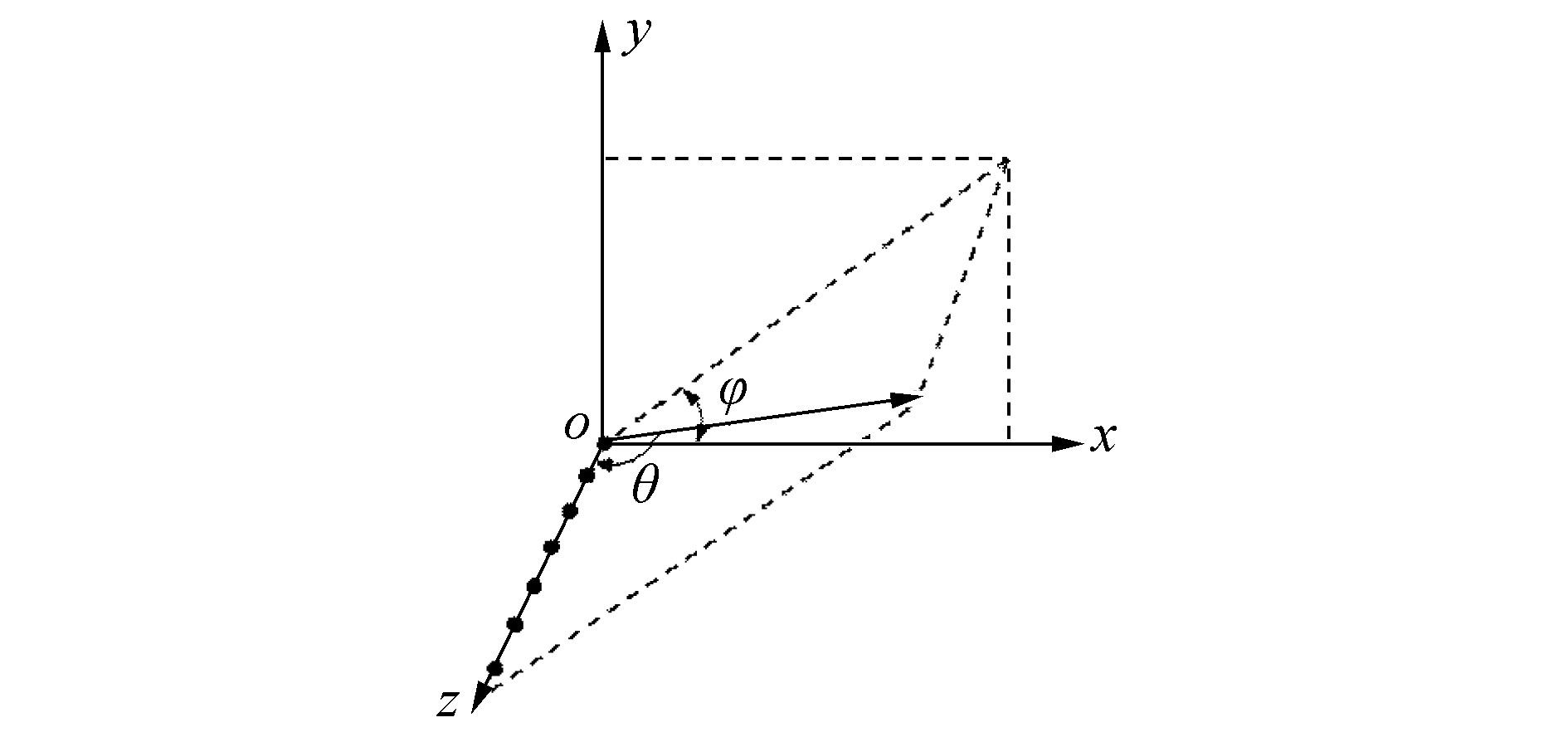Download: 图 1 矢量阵的几何模型 Fig. 1 Uniform line vector array geometry

 $P\left( {\theta ,\phi } \right) = \int_0^{2{\rm{ \mathsf{ π} }}} {\int_0^{\rm{ \mathsf{ π} }} {R\left( {\theta ,\phi \left| {\vartheta ,\varphi } \right.} \right)S\left( {\vartheta ,\varphi } \right)\sin \varphi {\rm{d}}\varphi {\rm{d}}\vartheta } }$ (5)

 $\begin{array}{*{20}{c}} {{R_V}\left( {\theta \left| \vartheta \right.} \right) = {{\left| {\frac{{\left( {1 + \cos \left( {\theta - \vartheta } \right)} \right)}}{2}} \right|}^2} \cdot }\\ {{{\left| {\frac{{\sin \left( {\frac{{N{\rm{ \mathsf{ π} }}d}}{\lambda }\left( {\cos \theta - \cos \vartheta } \right)} \right)}}{{N\sin \left( {\frac{{{\rm{ \mathsf{ π} }}d}}{\lambda }\left( {\cos \theta - \cos \vartheta } \right)} \right)}}} \right|}^2} = \mathit{\boldsymbol{U}}{\mathit{\boldsymbol{R}}_H}} \end{array}$ (6)

 $P\left( \theta \right) = \int_0^{2{\rm{ \mathsf{ π} }}} {{R_v}\left( {\theta \left| \vartheta \right.} \right)S\left( \vartheta \right)\sin \vartheta {\rm{d}}\vartheta }$ (7)

 $\begin{array}{*{20}{c}} {{R_V}\left( {\theta - \vartheta } \right) = {{\left| {\frac{{\left( {1 + \cos \left( {\theta - \vartheta } \right)} \right)}}{2}} \right|}^2} \cdot }\\ {{{\left| {\frac{{\sin \left( {\frac{{N{\rm{ \mathsf{ π} }}d}}{\lambda }\cos \left( {\theta - \vartheta } \right)} \right)}}{{N\sin \left( {\frac{{{\rm{ \mathsf{ π} }}d}}{\lambda }\cos \left( {\theta - \vartheta } \right)} \right)}}} \right|}^2}} \end{array}$ (8)

 $h\left( {t\left| \tau \right.} \right) \ne h\left( {t - \tau } \right)$ (9)

 $\begin{array}{l} y\left( t \right) = \int_{ - \infty }^{ + \infty } {h\left( {t\left| \tau \right.} \right)x\left( \tau \right){\rm{d}}\tau } = \\ \;\;\;\;\;\;\;\;\;\left( {{h_1}\left( {t\left| \tau \right.} \right){h_2}\left( {t\left| \tau \right.} \right)} \right) * x\left( t \right) \end{array}$ (10)

 $h\left( {t\left| \tau \right.} \right) = \left( {{h_1}\left( {t\left| \tau \right.} \right){h_2}\left( {t\left| \tau \right.} \right)} \right)$

1.4 NNLS反卷积求解方法

NNLS是一种在多个领域广泛应用的线性方程组求解方法。求解最小二乘问题，经典的方法是构造方程组，根据该方法求解的特点，可以将其应用于移变反卷积模型的求解。Ehrenfried利用NNLS求解噪声源定位的反卷积问题。褚志刚将该方法用于提高识别发动机噪声源的定位精度中。其基本原理可以推广到矢量阵反卷积处理，具体方法是在常规波束输出、矢量阵点扩散函数字典、声源目标函数之间建立差函数方程组，通过最小化差函数的原则来实现对目标函数S的求解，获取声源的分布，写做矩阵形式的表达式如下：

 $\mathit{\boldsymbol{\varphi }} = {\left\| {\mathit{\boldsymbol{RS}} - \mathit{\boldsymbol{P}}} \right\|_2}$ (11)

1) 由迭代得到的S(i)计算残差向量：

 $\boldsymbol{r}^{(i)}=\boldsymbol{R} \boldsymbol{S}^{(i)}-\boldsymbol{P}$ (12)

2) 计算ϕS空间的负梯度向量：

 $\boldsymbol{w}^{(i)}=-\boldsymbol{R}^{\mathrm{T}} \boldsymbol{r}^{(i)}$ (13)

3) 计算投影梯度w，定义搜索路径：

 ${\mathit{\boldsymbol{\bar w}}^{\left( i \right)}} = \left\{ \begin{array}{l} 0,\;\;\;\;\;\;\;{w^{\left( i \right)}} < 0,{\mathit{\boldsymbol{S}}^{\left( i \right)}} = 0\\ {\mathit{\boldsymbol{w}}^{\left( i \right)}},\;\;\;\;其他 \end{array} \right.$ (14)

4) 计算辅助向量：

 $\boldsymbol{g}^{(i)}=\boldsymbol{R} \overline{w}^{(i)}$ (15)

5) 计算最优搜索步长：

 $\lambda=-\frac{\boldsymbol{g}^{(i)} \cdot \boldsymbol{r}^{(i)}}{\boldsymbol{g}^{(i)} \cdot \boldsymbol{g}^{(i)}}$ (16)

6) 计算S(i+1)迭代结果：

 ${\mathit{\boldsymbol{S}}^{\left( {i + 1} \right)}} = \max \left( {{\mathit{\boldsymbol{S}}^{\left( i \right)}} + \lambda {{\bar w}^{\left( i \right)}},0} \right)$ (17)

NNLS算法并不局限于移不变反卷积模型求解，只需要获得阵列所有方位的指向性函数矩阵即可。需要注意的是，对于移不变模型采用NNLS算法时可采用FFT变换域计算的方式实现快速计算，但这并不适用于移变模型阵列。该方法并不局限于矢量阵，对结构固定不变的阵列该方法都适用。

2 仿真分析Download: 图 2 指向不同角度的矢量阵指向性函数 Fig. 2 The beam power distribution for a vector sensor line array for a signal at different degrees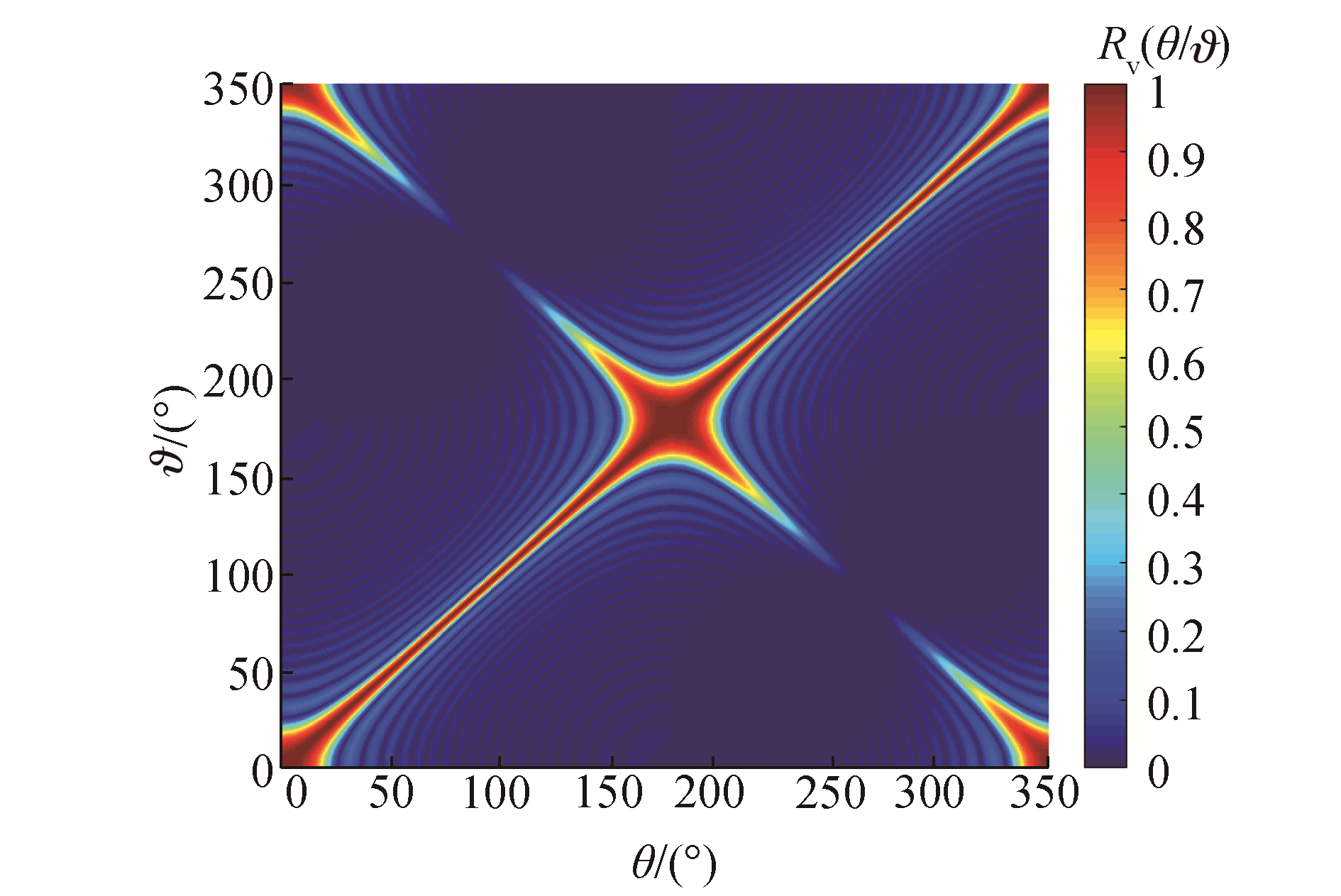Download: 图 3 预存PSF矩阵 Fig. 3 The predefined PSF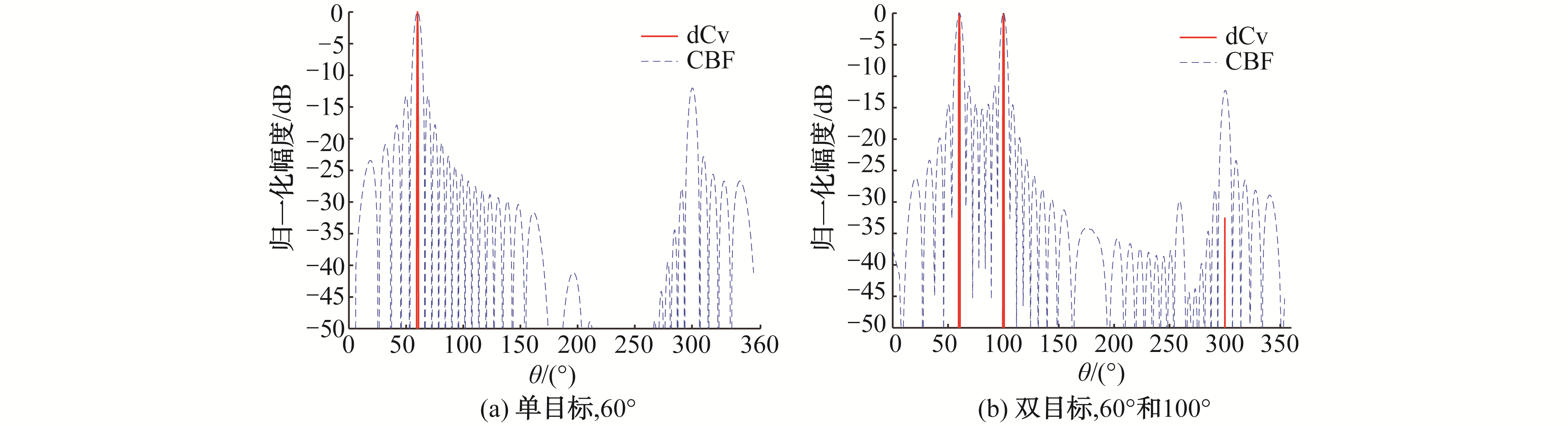Download: 图 4 矢量阵CBF和dCV的波束输出对比 Fig. 4 Comparison of dCv and CBF in vector array

2.1 阵增益仿真

 $\begin{array}{l} {\rm{DI}} = \frac{{4{\rm{ \mathsf{ π} }}\sigma _n^2}}{{\sigma _n^2\int_0^{\rm{ \mathsf{ π} }} {\int_{ - {\rm{ \mathsf{ π} }}}^{\rm{ \mathsf{ π} }} {R\left( {\theta ,\phi \left| {\vartheta ,\varphi } \right.} \right){\rm{d}}\varphi \sin \vartheta {\rm{d}}\vartheta } } }} = \\ \;\;\;\;\;\frac{4}{{\int_0^{{\rm{2 \mathsf{ π} }}} R (\theta |\vartheta )\sin \vartheta {\rm{d}}\vartheta }} \end{array}$ (18)

 ${\rm{DG}} = \frac{{{\rm{D}}{{\rm{I}}_{{\rm{dGv}}}}}}{{{\rm{D}}{{\rm{I}}_{{\rm{CBF}}}}}} = \frac{{\int_0^{\rm{ \mathsf{ π} }} {{R_{{\rm{CBF}}}}\left( {\theta \left| \vartheta \right.} \right)\sin \vartheta {\rm{d}}\vartheta } }}{{\int_{ - {\rm{ \mathsf{ π} }}}^{\rm{ \mathsf{ π} }} {{R_{{\rm{dCv}}}}\left( {\theta \left| \vartheta \right.} \right)\sin \vartheta {\rm{d}}\vartheta } }}$ (19)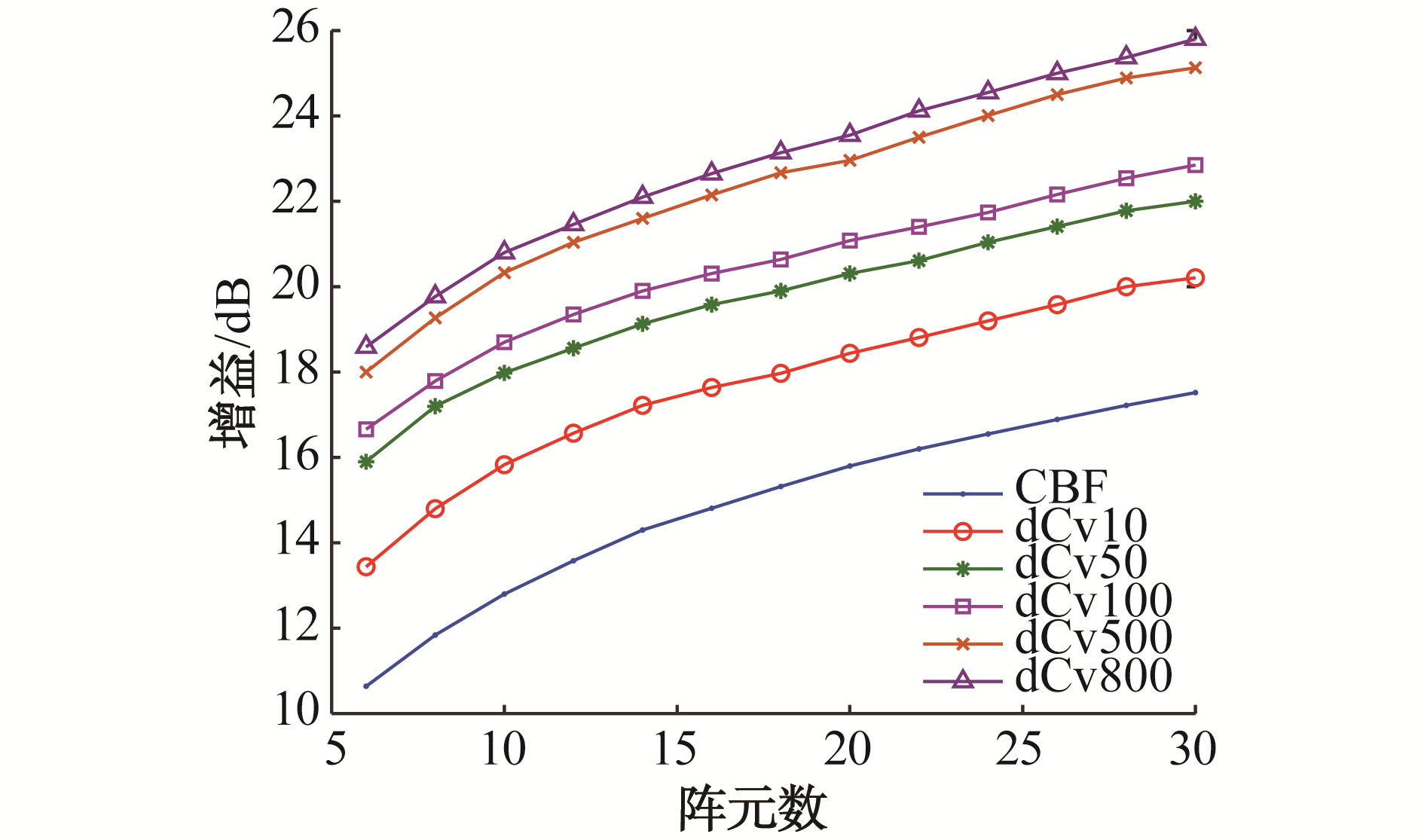Download: 图 5 矢量阵CBF和dCV的增益对比分析 Fig. 5 Directivity index comparison of CBF and dCV as a function of the number of elements
2.2 矢量阵左右舷抑制能力对比仿真

 $G\left( {\theta ,{\theta _0}} \right) = 10\lg {\left| {\frac{{R\left( {\theta ,{\theta _0}} \right)}}{{R\left( { - \theta ,{\theta _0}} \right)}}} \right|^2}$ (20)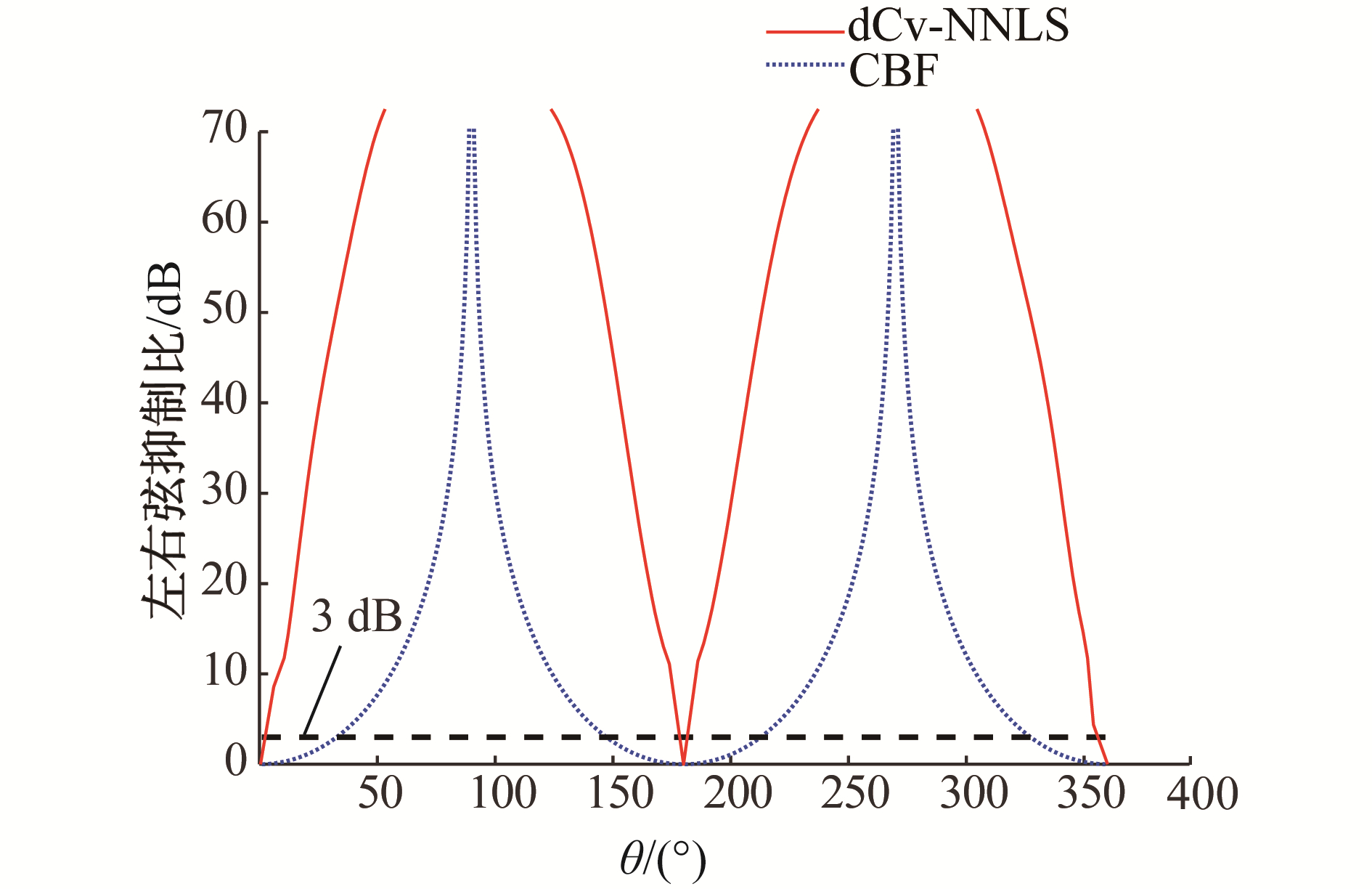Download: 图 6 矢量阵CBF和dCV的左右舷抑制比 Fig. 6 The left/right suppression ratio of dCv and CBF

2.3 矢量阵反卷积与多种波束形成算法性能对比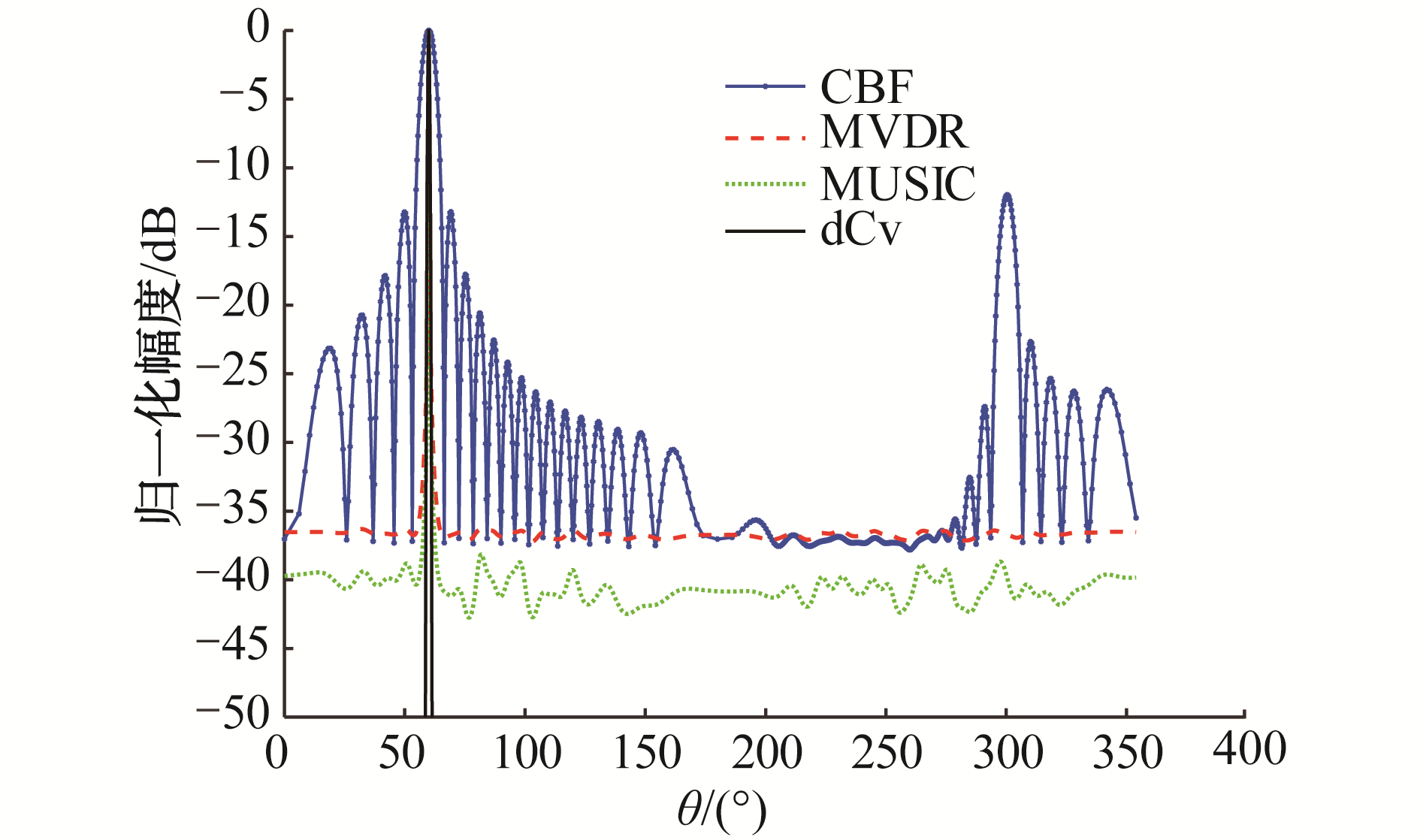Download: 图 7 矢量阵波束输出空间谱DOA估计性能比较 Fig. 7 Beam power distribution using different DOA algorithms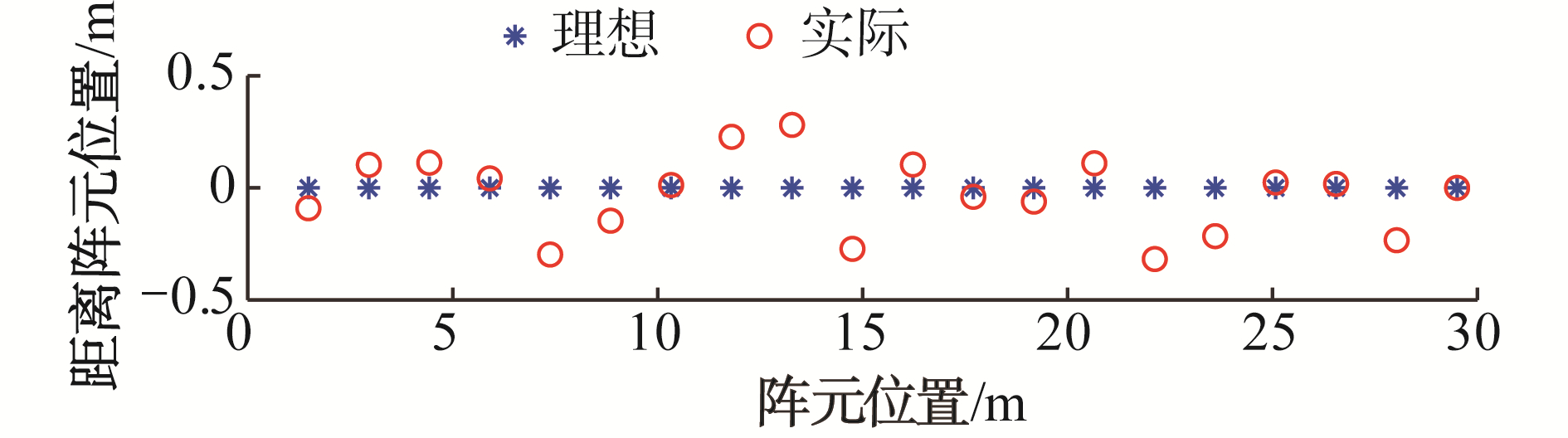Download: 图 8 无阵元位置与有阵元位置对比 Fig. 8 The array configuration with and without position error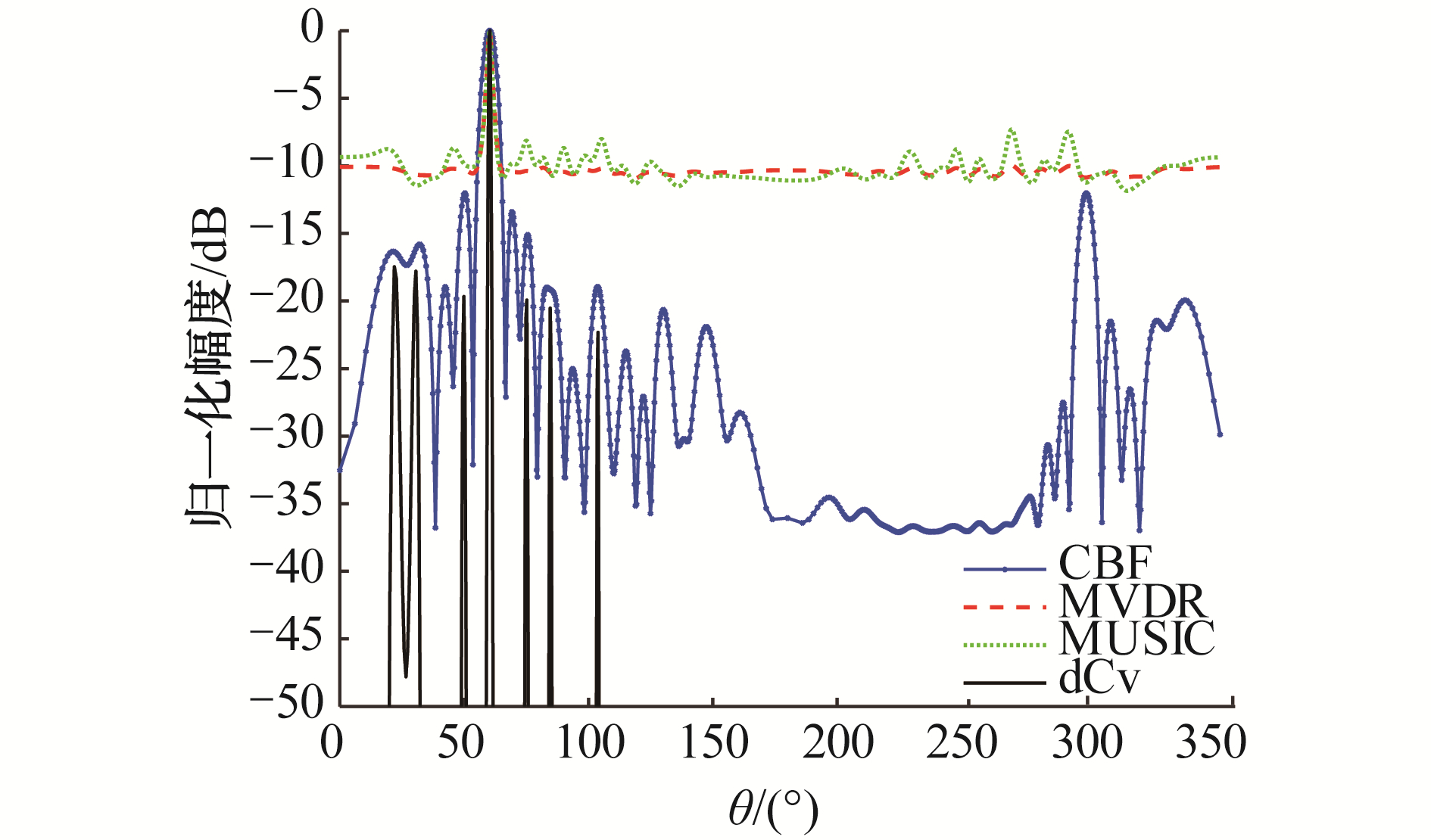Download: 图 9 有阵元位置误差时矢量阵CBF和dCV的波束输出对比 Fig. 9 Beam output power comparison of CBF and dCV with array position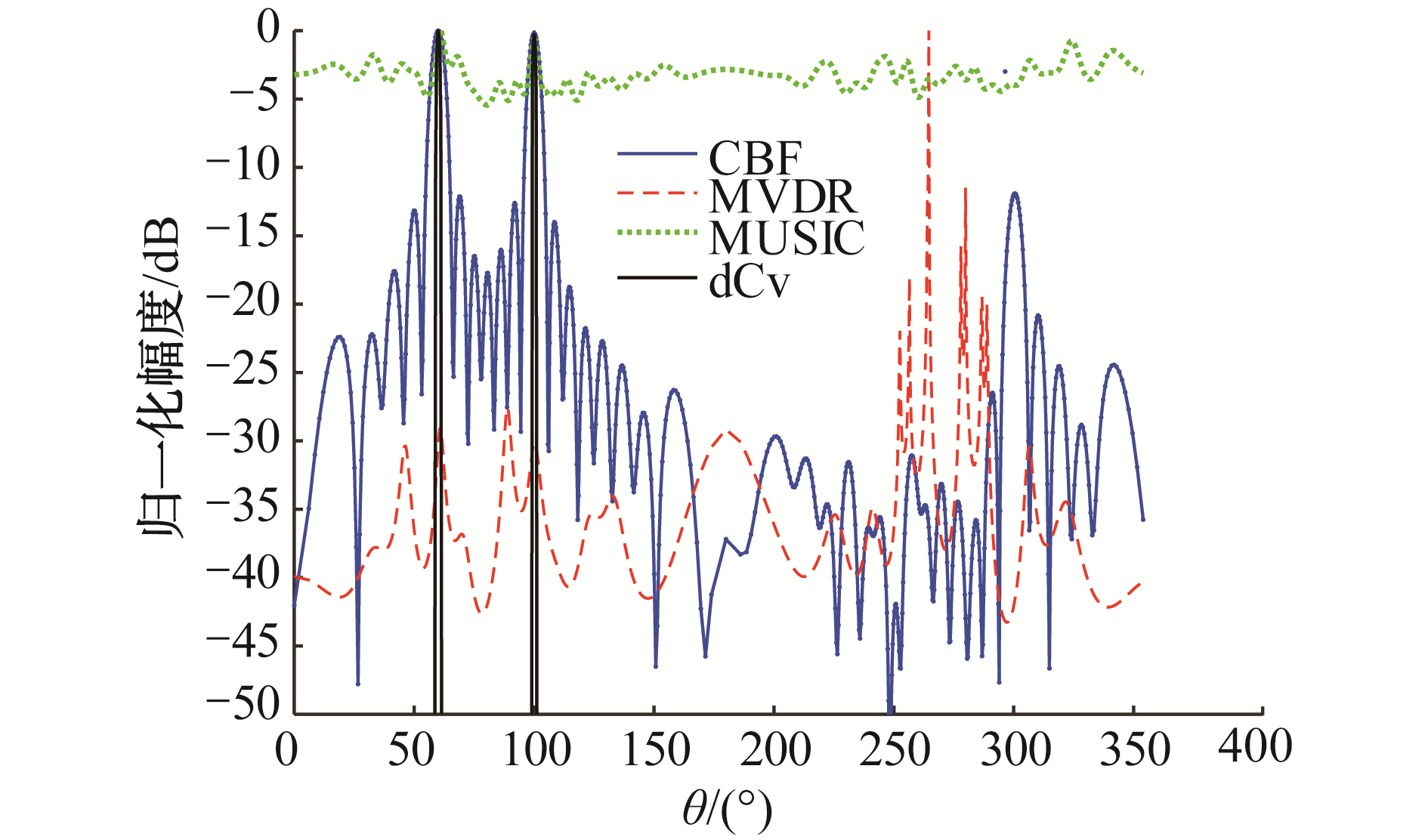Download: 图 10 单快拍波束输出空间谱估计性能比较 Fig. 10 Beam output power comparison for single snapshot data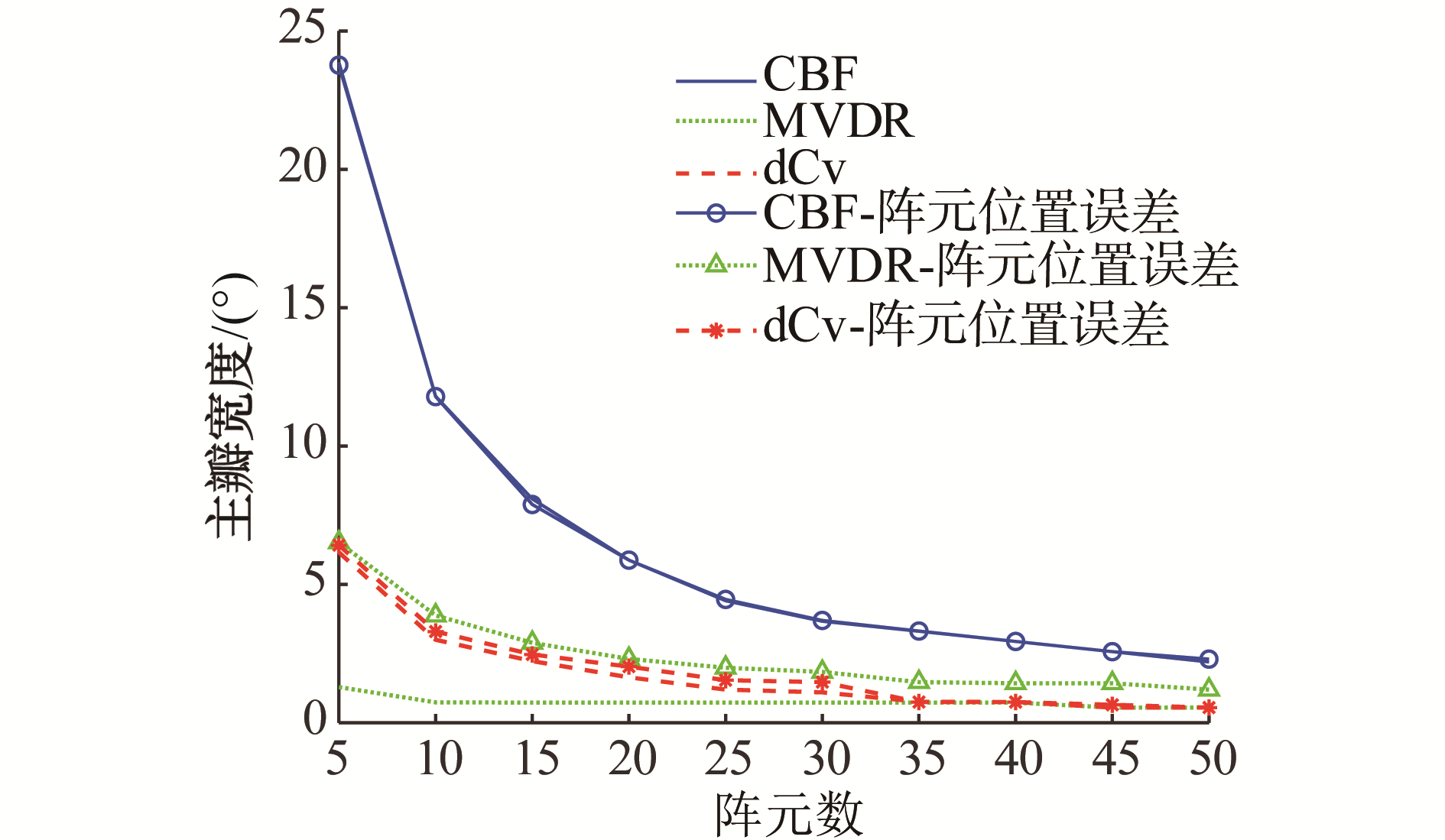Download: 图 11 不同阵元数的主瓣宽度对比图 Fig. 11 Beam width as a function of the number of elements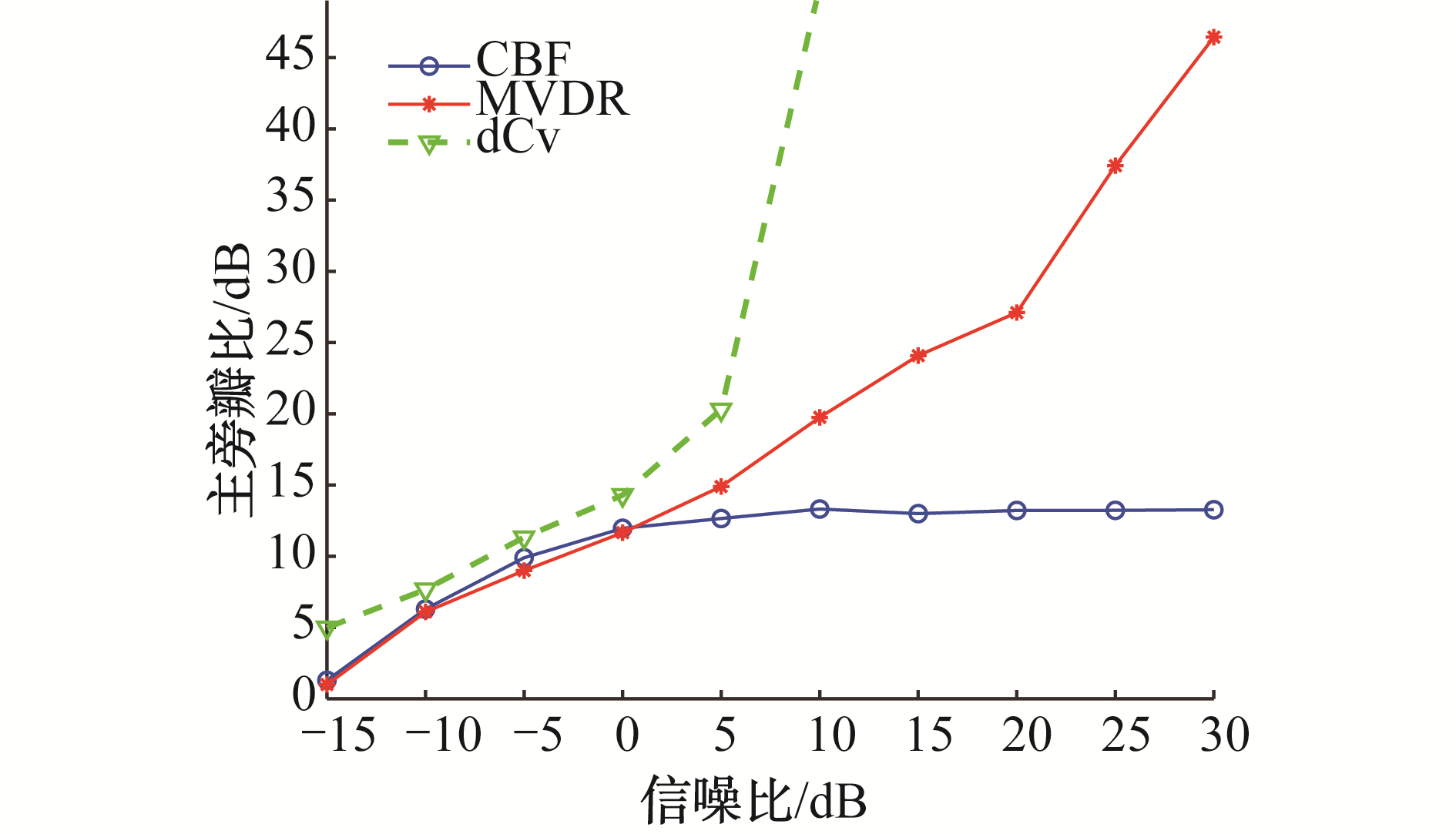Download: 图 12 不同输入信噪比下的主旁瓣对比 Fig. 12 The peak-to-sidelobe ratio as a function of input SNR
3 结论

1) 针对固定阵的阵列信号处理模型PSF可预测的特点，将噪声源定位中的非负最小二乘方法应用到矢量阵中，使反卷积波束形成算法可以应用到PSF是移变的阵列模型中。

2) 以矢量阵为例仿真证明了该算法的可行性，仿真结果表明，方法与矢量阵CBF和其他高分辨算法相比具有更好的增益、更窄的主瓣和低的旁瓣，且适用于快拍数少和存在阵元位置误差的情况下，具有良好的稳健性。

  DE CASTRO F C F, DE CASTRO M C F, ARANTES D S. Concurrent blind deconvolution for channel equalization[C]//Proceedings of IEEE International Conference on Communications. Helsinki, Finland, 2001: 366-371. https://ieeexplore.ieee.org/document/936964/ (0)  NSIRI B, CHONAVEL T, BOUCHER J M, et al. Blind submarine seismic deconvolution for long source wavelets[J]. IEEE journal of oceanic engineering, 2007, 32(3): 729-743. DOI:10.1109/JOE.2007.899408 (0)  LYLLOFF O, FERNÃNDEZ-GRANDE E, AGERKVIST F, et al. Improving the efficiency of deconvolution algorithms for sound source localization[J]. The journal of the acoustical society of America, 2015, 138(1): 172-180. DOI:10.1121/1.4922516 (0)  ZHONG Di, YANG Dong, ZHU Min. Improvement of sound source localization in a finite duct using beamforming methods[J]. Applied acoustics, 2016, 103: 37-46. DOI:10.1016/j.apacoust.2015.10.007 (0)  VONESCH C, UNSER M. A fast thresholded landweber algorithm for wavelet-regularized multidimensional deconvolution[J]. IEEE transactions on image processing, 2008, 17(4): 539-549. (0)  SEKKO E, THOMAS G, BOUKROUCHE A. A deconvolution technique using optimal Wiener filtering and regularization[J]. Signal processing, 1999, 72(1): 23-32. (0)  DOUGHERTY R. Extensions of DAMAS and benefits and limitations of deconvolution in beamforming[C]//Proceedings of the 11th AIAA/CEAS Aeroacoustics Conference. Monterey, California, 2005. https://www.researchgate.net/publication/260624421_Extensions_of_DAMAS_and_Benefits_and_Limitations_of_Deconvolution_in_Beamforming (0)  EHRENFRIED K, KOOP L. Comparison of iterative deconvolution algorithms for the mapping of acoustic sources[J]. AIAA journal, 2012, 45(7): 1584-1595. (0)  BLAHUT R E. Theory of remote image formation[M]. Cambridge: Cambridge University Press, 2004. (0)  YANG T C. Deconvolved conventional beamforming for a horizontal line array[J]. IEEE journal of oceanic engineering, 2018, 43(1): 160-172. DOI:10.1109/JOE.2017.2680818 (0)  YANG T C. Performance analysis of superdirectivity of circular arrays and implications for sonar systems[J]. IEEE journal of oceanic engineering, 2019, 44(1): 156-166. DOI:10.1109/JOE.2018.2801144 (0)  梁国龙, 马巍, 范展, 等. 矢量声纳高速运动目标稳健高分辨方位估计[J]. 物理学报, 2013, 62(14): 144302. LIANG Guolong, MA Wei, FAN Zhan, et al. A high resolution robust localization approach of high speed target based on vector sonar[J]. Acta physica sinica, 2013, 62(14): 144302. DOI:10.7498/aps.62.144302 (0)  时洁, 杨德森, 时胜国. 基于最差性能优化的运动声源稳健聚焦定位识别方法研究[J]. 物理学报, 2011, 60(6): 064301. SHI Jie, YANG Desen, SHI Shengguo. Robust localization and identification method of moving sound sources based on worst-case performance optimization[J]. Acta physica sinica, 2011, 60(6): 064301. (0)  褚志刚, 杨洋. 基于非负最小二乘反卷积波束形成的发动机噪声源识别[J]. 振动与冲击, 2013, 32(23): 75-81. CHU Zhigang, YANG Yang. Noise source identification for an engine based on FFT-non-negative least square (NNLS) deconvolution beamforming[J]. Journal of vibration and shock, 2013, 32(23): 75-81. DOI:10.3969/j.issn.1000-3835.2013.23.014 (0)  张宾, 孙贵青, 李启虎. 阵形畸变对拖曳双线阵左右舷分辨性能的影响[J]. 声学学报, 2008, 33(4): 294-299. ZHANG Bin, SUN Guiqing, LI Qihu. Effects of shape distortion upon left/right discrimination of towed twin-line array[J]. Acta acustica, 2008, 33(4): 294-299. DOI:10.3321/j.issn:0371-0025.2008.04.002 (0)